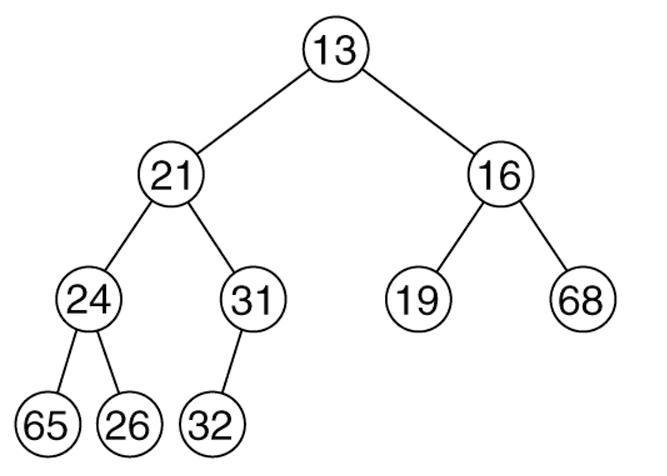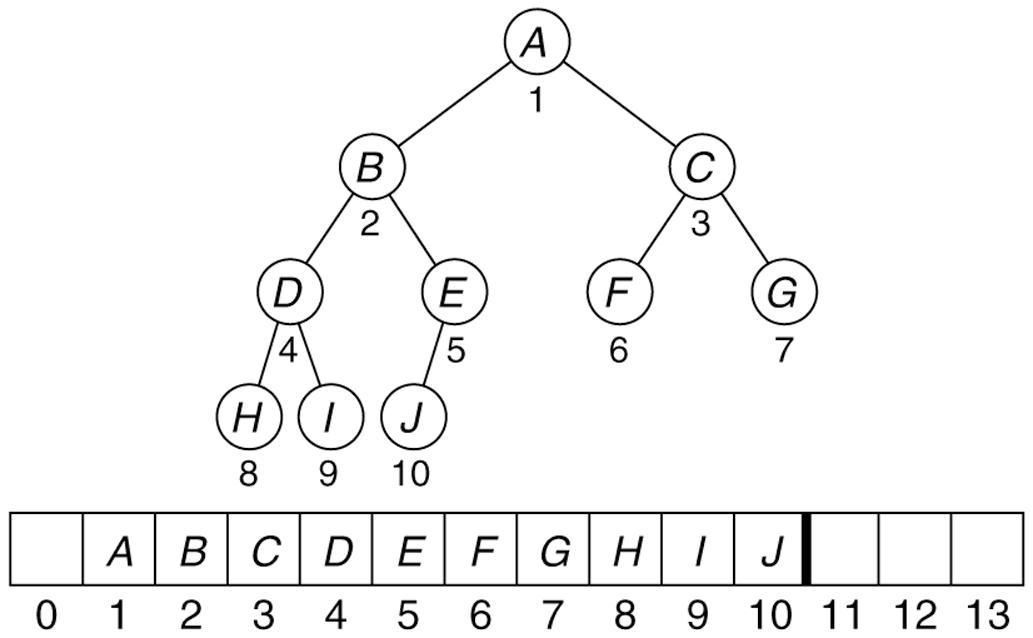## HEAP (Min Heap)

Ok its been a long time i have posted here , but the Data Structure i am
gonna discuss today is one of the most frequently used Data Structure . Its called a HEAP or a priority queue . Its a DS in which the data is stored
depending on the priority set by the user.
So the fact i am gonna use here is that
the element at the root  should be less than both of its left and right child
and the binary should be a complete binary tree.So to implement such kind of a DS we actually do not need to implement the binary tree , we can make a wise use of array as :Inserting an element:
• In the Shuffleup operation, the value of a position,is compared with that the value of its parent.
• If the value is smaller than its parent, then they are swapped.
• This is done until either value is bigger than the value at its parent or we have reached the root.
Deleting an Element:

• This is done by first relocating it to the root,satisfying the complete binary tree property.
• This relocation may however violate the heap invariant.
• To restore the heap invariant, we perform what is known as Shuffle-Down.
Shuffle Down:

• In Shuffle-Down, analogous to Shuffle-Up, we move the element down the tree until its value is at most the value of any of its children.
• Every time the value at a node X is bigger than the value of either of its children, the smallest child and X are swapped.
Explanation for the Code:
I x ( insert integer x in heap )
D ( Delete the minimum element from heap )
P ( Print the heap )

comments powered by Disqus